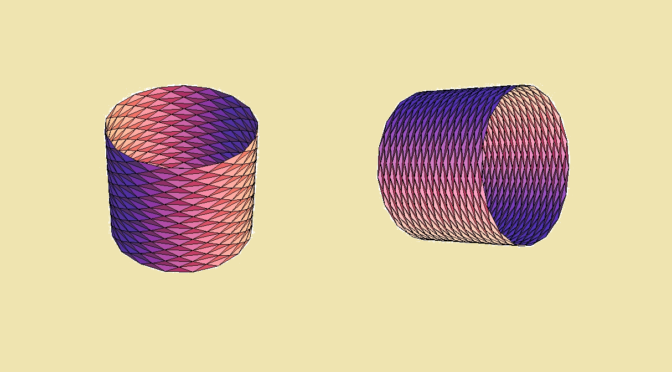# The Schwarz lantern

Consider a smooth curve defined by a continuous map $$f : [0,1] \to \mathbb R^n$$ with $$n \ge 2$$ where $$f$$ is supposed to have a continuous derivative. One can prove that the curve is rectifiable, its arc length being $L = \lim\limits_{n \to \infty} \sum_{i=1}^n \vert f(t_i) – f(t_{i-1}) \vert = \int_0^1 \vert f^\prime (t) \vert \ dt$ with $$t_i = \frac{i}{n}$$ for $$0 \le i \le n$$.

What can happen when we consider a surface instead of a curve?

Consider a compact, smooth surface (possibly with boundary) embedded in $$\mathbb R^3$$. We can approximate it as a polyhedral surface composed of small triangles with all vertices on the initial surface. Will the sum of the areas of the triangles converges to the area of the surface if their size is converging to zero?

The answer is negative and we provide a counterexample named Schwarz lantern. We take a cylinder of radius $$r$$ and height $$h$$. We approximate the cylinder by $$4nm$$ isosceles triangles positioned as in the picture in $$2n$$ slices. All triangles have the same base and height given by $b = 2r \sin \left(\frac{\pi}{m}\right), \ h = \sqrt{r^2 \left[1-\cos \left(\frac{\pi}{m}\right)\right]^2+\left(\frac{h}{2n}\right)^2}$ Hence the area of the polyhedral surface is \begin{aligned} S^\prime(m,n) &= 4 m n r \sin \left(\frac{\pi}{m}\right) \sqrt{r^2 \left[1-\cos \left(\frac{\pi}{m}\right)\right]^2+\left(\frac{h}{2n}\right)^2}\\ &= 4 m n r \sin \left(\frac{\pi}{m}\right) \sqrt{4 r^2 \sin^4 \left(\frac{\pi}{2m} \right)+\left(\frac{h}{2n}\right)^2} \end{aligned} From there, let’s have a look to the value of $$S^\prime(m,n)$$ as $$m,n \to \infty$$.

#### Suppose that $$n=am$$ with $$a >0$$

In that case we have $\lim\limits_{m \to \infty} S^\prime(m,am) = S = 2 \pi r h$ where $$S$$ is the area of the cylinder. We are in a similar case to the one of a smooth curve.

#### Now suppose that $$n=am^2$$ with $$a >0$$

Then $\lim\limits_{m \to \infty} S^\prime(m,am^2) = 2 \pi r \sqrt{a^2 \pi^2 r^2 + h^2}$ The limit area of the polyhedral surface is finite, but can be made arbitrarily larger than the one of the cylinder as $$a$$ is increasing.

#### Finally, let’s take $$n=m^3$$

We have $S^\prime(m,m^3) \ge 8 r^2 m^4 \sin \left(\frac{\pi}{m}\right) \sin^2 \left(\frac{\pi}{2m}\right)$ In that case $\lim\limits_{m \to \infty} S^\prime(m,m^3) = \infty.$

Conclusion: the area of the cylinder cannot be measured as the limit area of a polyhedral surface composed of triangles if we don’t impose appropriate conditions on the triangles shape.